# Resources

Resources - Haymik Math HelpersNew and innovative math resources for the 21st century, called the “Haymik Math Helpers” each product, specifically designed for a succession of learning experiences, from pre- schoolers to years ten or eleven or for older students who struggle with maths. Using these helpers children can use both manipulative and visualisation processes to gain both knowledge and an understanding of a mathematical concept. Resources that help children to initially gain a basic overall understanding of a mathematical concept and then to progress to a more sophisticated overall understanding of strategies and techniques needed to solve problems accurately and efficiently.Representation and visualisation are the two of the most powerful tools to assist students to learn by discovery, gaining both knowledge and an understanding of mathematical concepts such as:  The relationships between numbers and the quantities for which they stand, visualising the act of addition, subtraction, multiplication, and fractions and to succeed in these operations. This process helps students to develop the ability to think and reason logically, visualising a variety of approaches and clever strategies to solve mathematical problems gaining accuracy, efficiency and confidence.

Our new products have been especially designed, using the latest research and information to impart knowledge and skills to equip our children for the 21st century.
The method used is called Velcro learning because, it requires two parts, Representation and Visualisation the two of the  most powerful tools to assist students to learn by discovery, gaining both knowledge and then to synthesise it with what they already know to construct their own understanding of mathematical concepts.
• ### Haymik Maths Helper 10 in both English and Bilingual version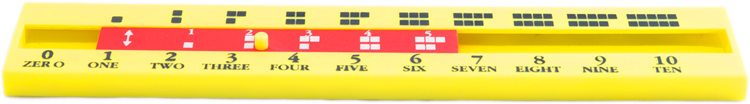The 10 Frame’s helper assist children to develop an understanding of number, the way they are represented and the quantities for which they stand, Counting on, ordering, and comparing numbers up to 5 (and later up to 9).
• ### Haymik 20 number line slide rule in English and Bilingual version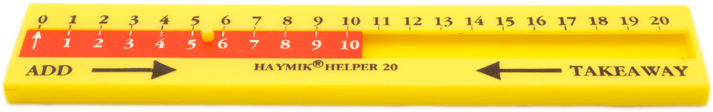The benefits are its unique number line concept and visualisation process, that helps children form easy and clever strategies to solve mathematical problems, and improve their math skills. Following up on requests for Maori resources, we have designed a bilingual version.
• ### Haymik Helper 30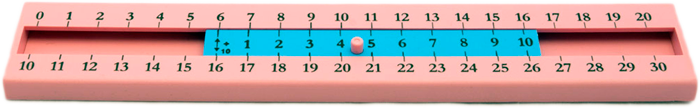###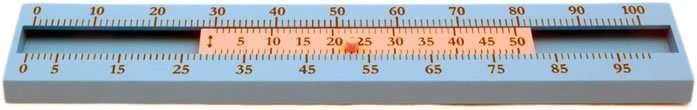Takes children passed 20, to the next level in their number development and shows them how to work with two or more digit numbers, learning place value (tens notation), for which the Haymik Helper 30 has been especially designed. Assists children to develop a wider variety of approaches to solving mathematical problems, building confidence and enjoyment for a continuing and creative interest in maths.  The H100 takes children up another level again, skip counting by 5’s and 10’s. Learning slide rule strategies and clever techniques to enhance their math skills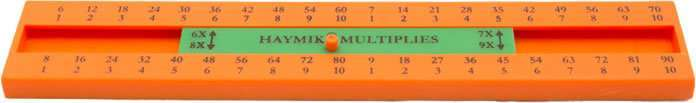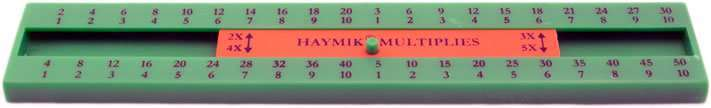• ### Haymik Multipliers. ( 2 )

This set of Haymik Multipliers is to help children explore, by way of discovery, how the number system is made up. Assists them as a prelude to learning their times tables, to count up in sets i.e.. count in two’s, three’s, four’s etc.  The Haymik Multipliers are not only a fun way to learn their time tables, but helps them visualise multiplication as successive addition and aids children to think more cleverly and efficiently about numbers.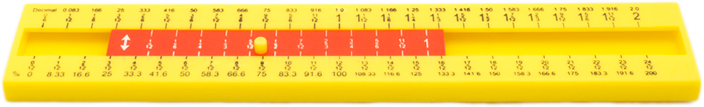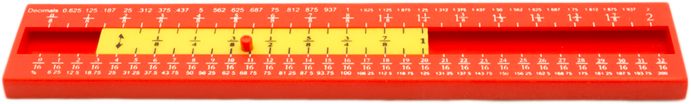• ### Haymik Fraction Helpers ( 2 )

Fractions on the number line slide rule helps children compare the relative sizes of fractions, and their equivalence.  Assists students visualise the act of adding and subtracting fractions and to succeed in these operations.
Help with Fractions and their conversion to decimals and percentages.
Assists children to examine relationships between fractions and decimals and learn addition and subtraction facts.
Unique design to help children explore and discover numbers gaining both knowledge and competence

## Techniques and strategies related to addition and subtraction include:

• Counting (counting on or back)
A useful strategy when counting on or back 1, 2 or 3 numbers.
• Commutativity
This refers to the strategy of reversing numbers so that the larger is first.
E.g.. 2 + 7 to 7 + 2   (2 + 7 = 7 + 2)
• Skip counting by 2, 3, and 5 form 0 and 1
Prelude to learning their times tables and helps to find doubles
• Doubles
Using doubles and near doubles.
E.g.. 7 + 8 = (7 + 7 = 14 + 1 more is 15) or (8 + 8 = 16 less 1 = 15)
• Reversibility
Turning a subtraction into an addition or an addition into a subtraction.
E.g.. 13 – 6 = ? to 6 + ? = 13
Or 7 + 5 = ?   to ? – 5 = 7